# Squeeze Theorem: Definition and Examples

An error occurred trying to load this video.

Try refreshing the page, or contact customer support.

Coming up next: Graphs and Limits: Defining Asymptotes and Infinity

### You're on a roll. Keep up the good work!

Replay
Your next lesson will play in 10 seconds
• 0:06 Intro to the Squeeze Theorem
• 1:22 Understanding the…
• 2:56 Squeeze Theorem in Practice
• 5:04 Lesson Summary
Save Save

Want to watch this again later?

Timeline
Autoplay
Autoplay
Speed Speed Audio mode

#### Recommended Lessons and Courses for You

Lesson Transcript
Instructor
Jeff Calareso

Jeff teaches high school English, math and other subjects. He has a master's degree in writing and literature.

Expert Contributor
Simona Hodis

Simona received her PhD in Applied Mathematics in 2010 and is a college professor teaching undergraduate mathematics courses.

In the Kingdom of Rimonn there are three rivers. In this lesson, learn how these waterways demonstrate the power of the squeeze theorem for finding the limits of functions.

## Introduction to the Squeeze Theorem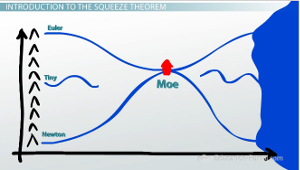Welcome to the Kingdom of Rimonn! Now in the Kingdom of Rimonn, we have three primary rivers. We have the River Euler. We have the River Newton. And we have the River Tiny. We don't exactly know where Tiny goes, but we know he starts out in the hills and he ends in the sea. We know a few things about the rivers in the Kingdom of Rimonn. We know that Euler is always north of the River Newton. We know that Tiny is always north of Newton, but south of Euler. So we know that Tiny basically is always between Newton and Euler, we just don't know exactly where he goes.

We also know that Euler and Newton meet up. They get very close in a village called Moe. So because Tiny is surrounded by Newton and Euler throughout the entire length of the river, we know that Tiny also has to meet up in the town of Moe. Because we know that Tiny doesn't cross Euler or Newton, and since they meet up at Moe, Tiny must also meet up at Moe.

## Understanding the Squeeze Theorem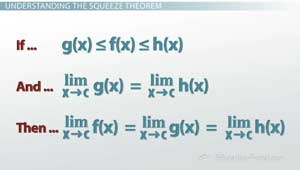This principle is known as the squeeze theorem in calculus. Some people call it the sandwich theorem, but I like the term squeeze.

Now let's consider the village of Moe, and let's zoom in really close where Euler and Newton meet up. I can say that the limit, as we approach Moe, of Euler is this point here. Let's call it the town square. And the limit, as we approach Moe, of Newton is also the town square. Because Euler is always north of Tiny and Tiny is always north of Newton, I can write that the limit, as we approach Moe, of Tiny is also the town square.

So let's make this really formal. If the function g(x) is less than or equal to f(x), which is less than or equal to h(x), and the limit, as we approach some number, of g(x) equals the limit, as we approach that same number, of h(x), then we've squeezed f(x) such that the limit, as we approach the same number, of f(x) is equal to both the limit of both g and h. In this case, h is like Euler, g is like Newton and f is like Tiny, and f is squeezed in here. So the limit as we approach Moe is that town square.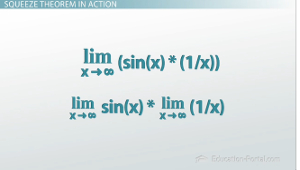To unlock this lesson you must be a Study.com Member.

### Review Terms

The Squeeze Theorem is a technique to evaluate the limit of a function by finding two functions that bound the given function below and above and the two functions approach the same finite value when the limits are evaluated.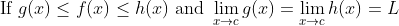Then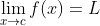Squeeze Theorem is usually used when we have sine or cosine terms because they are bounded by -1 and 1.

### Application - Limits in Two Variables

For example, the limit of a function of two variables at a point (a, b) is the finite value, L, such that, for any disk centered at (a, b), whose radius goes to zero (so the disk approaches its center), the function approaches the unique value L: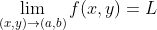A disk can be represented in polar coordinates as: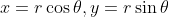So a two-dimensional limit can be written as a one-dimensional limit.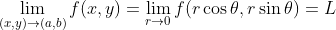Usually, the limit in polar coordinates is evaluated with the Squeeze Theorem.

### Application

To evaluate the limits below, convert them to polar coordinates and evaluate.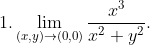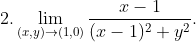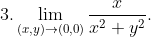### Solutions

1. Using the polar coordinates centered at the origin and radius r: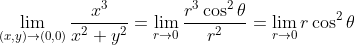Because the cosine term is bounded by -1 and 1, the square of cosine is bounded by 0 and 1, so: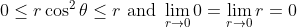And by the Squeeze Theorem, the limit is zero.

2. Using the polar coordinates centered at the point, (1, 0), and radius, r: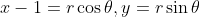the limit is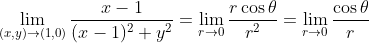which does not exist.

3. Using the polar coordinates centered at the origin and radius r, we obtain: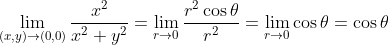This does not exist because the limit depends on theta, so the limit will not be unique, it will be different for different values of theta.

### Register to view this lesson

Are you a student or a teacher?

#### See for yourself why 30 million people use Study.com

##### Become a Study.com member and start learning now.
Back
What teachers are saying about Study.com

### Earning College Credit

Did you know… We have over 200 college courses that prepare you to earn credit by exam that is accepted by over 1,500 colleges and universities. You can test out of the first two years of college and save thousands off your degree. Anyone can earn credit-by-exam regardless of age or education level.